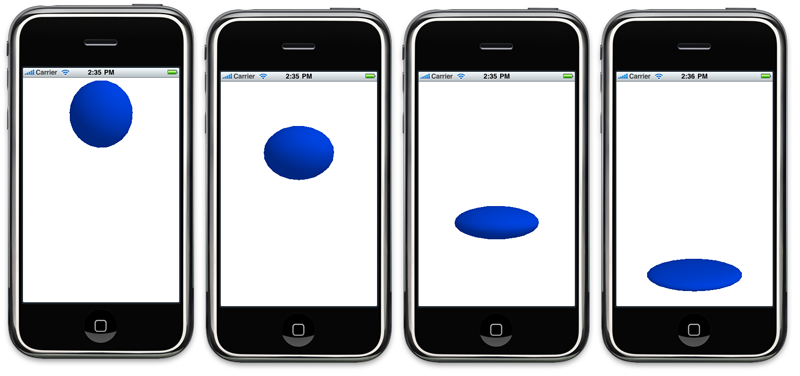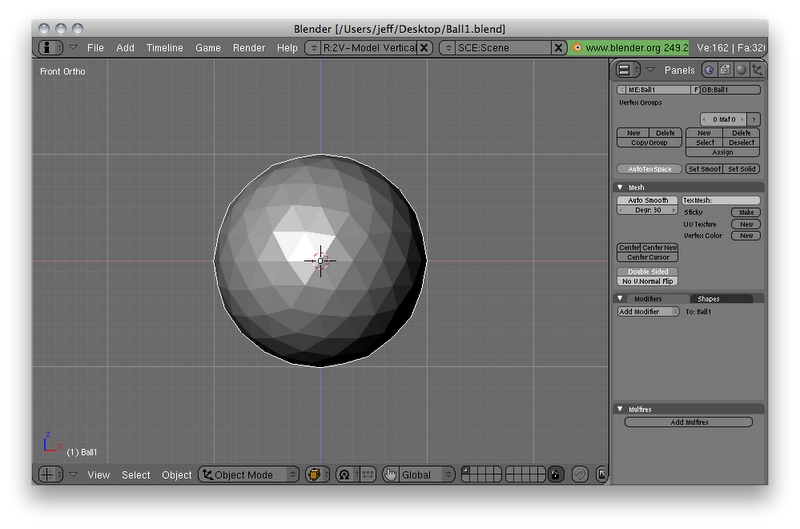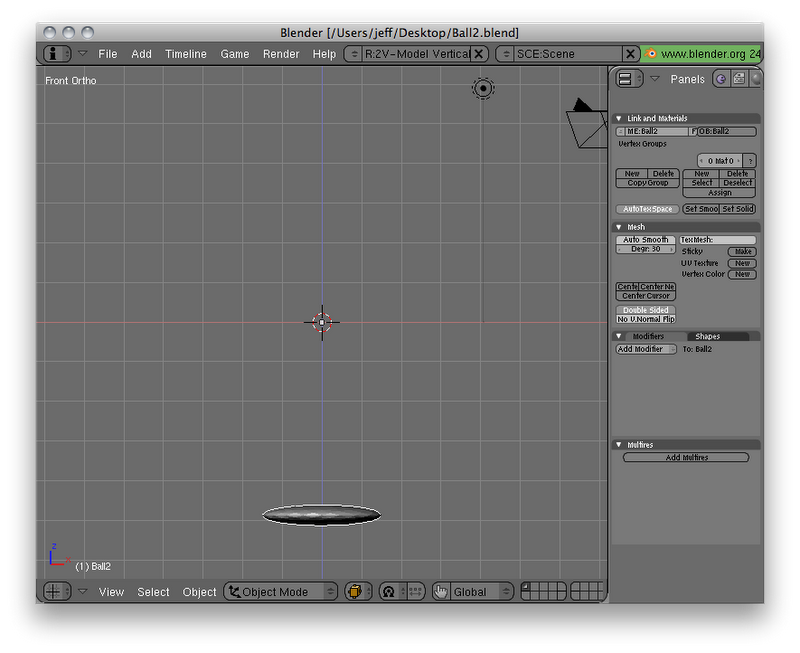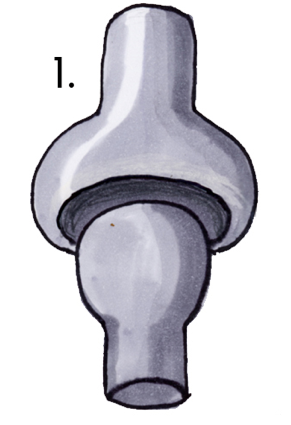# OpenGL ES 从零开始系列9：动画基础和关键帧动画

### Keyframe Animation in OpenGLES#define kAnimationDuration  0.3
enum animationDirection {
kAnimationDirectionForward = YES,
kAnimationDirectionBackward = NO
};
typedef BOOL AnimationDirection;


- (void)drawView:(UIView *)theView
{
static NSTimeInterval lastKeyframeTime = 0.0;
if (lastKeyframeTime == 0.0)
lastKeyframeTime = [NSDate timeIntervalSinceReferenceDate];
static AnimationDirection direction = kAnimationDirectionForward;

glClearColor(1.0, 1.0, 1.0, 1.0);
glClear(GL_COLOR_BUFFER_BIT | GL_DEPTH_BUFFER_BIT);
glLoadIdentity();
glTranslatef(0.0f,2.2f,-6.0f);
glRotatef(-90.0, 1.0, 0.0, 0.0); // Blender uses Z-up, not Y-up like OpenGL ES

static VertexData3D ballVertexData[kBall1NumberOfVertices];

glColor4f(0.0, 0.3, 1.0, 1.0);
glEnable(GL_COLOR_MATERIAL);
NSTimeInterval timeSinceLastKeyFrame = [NSDate timeIntervalSinceReferenceDate]                                                                          - lastKeyframeTime;
if (timeSinceLastKeyFrame > kAnimationDuration) {
direction = !direction;
timeSinceLastKeyFrame = timeSinceLastKeyFrame - kAnimationDuration;
lastKeyframeTime = [NSDate timeIntervalSinceReferenceDate];
}
NSTimeInterval percentDone = timeSinceLastKeyFrame / kAnimationDuration;

VertexData3D *source, *dest;
if (direction == kAnimationDirectionForward)
{
source = (VertexData3D *)Ball1VertexData;
dest = (VertexData3D *)Ball2VertexData;
}
else
{
source = (VertexData3D *)Ball2VertexData;
dest = (VertexData3D *)Ball1VertexData;
}

for (int i = 0; i < kBall1NumberOfVertices; i++)
{
GLfloat diffX = dest[i].vertex.x - source[i].vertex.x;
GLfloat diffY = dest[i].vertex.y - source[i].vertex.y;
GLfloat diffZ = dest[i].vertex.z - source[i].vertex.z;
GLfloat diffNormalX = dest[i].normal.x - source[i].normal.x;
GLfloat diffNormalY = dest[i].normal.y - source[i].normal.y;
GLfloat diffNormalZ = dest[i].normal.z - source[i].normal.z;

ballVertexData[i].vertex.x = source[i].vertex.x + (percentDone * diffX);
ballVertexData[i].vertex.y = source[i].vertex.y + (percentDone * diffY);
ballVertexData[i].vertex.z = source[i].vertex.z + (percentDone * diffZ);
ballVertexData[i].normal.x = source[i].normal.x + (percentDone * diffNormalX);
ballVertexData[i].normal.y = source[i].normal.y + (percentDone * diffNormalY);
ballVertexData[i].normal.z = source[i].normal.z + (percentDone * diffNormalZ);

}

glEnableClientState(GL_VERTEX_ARRAY);
glEnableClientState(GL_NORMAL_ARRAY);
glVertexPointer(3, GL_FLOAT, sizeof(VertexData3D), &Ball2VertexData.vertex);
glNormalPointer(GL_FLOAT, sizeof(VertexData3D), &Ball2VertexData.normal);
glDrawArrays(GL_TRIANGLES, 0, kBall1NumberOfVertices);
glDisableClientState(GL_VERTEX_ARRAY);
glDisableClientState(GL_NORMAL_ARRAY);
}


    static NSTimeInterval lastKeyframeTime = 0.0;
if (lastKeyframeTime == 0.0)
lastKeyframeTime = [NSDate timeIntervalSinceReferenceDate];
static AnimationDirection direction = kAnimationDirectionForward;


    glClearColor(1.0, 1.0, 1.0, 1.0);
glClear(GL_COLOR_BUFFER_BIT | GL_DEPTH_BUFFER_BIT);
glLoadIdentity();
glTranslatef(0.0f,2.2f,-6.0f);
glRotatef(-90.0, 1.0, 0.0, 0.0); // Blender uses Z-up, not Y-up like OpenGL ES


    static VertexData3D ballVertexData[kBall1NumberOfVertices];


    glColor4f(0.0, 0.3, 1.0, 1.0);
glEnable(GL_COLOR_MATERIAL);


    NSTimeInterval timeSinceLastKeyFrame = [NSDate timeIntervalSinceReferenceDate]                                                                                                   - lastKeyframeTime;
if (timeSinceLastKeyFrame > kAnimationDuration) {
direction = !direction;
timeSinceLastKeyFrame = timeSinceLastKeyFrame - kAnimationDuration;
lastKeyframeTime = [NSDate timeIntervalSinceReferenceDate];
}
NSTimeInterval percentDone = timeSinceLastKeyFrame / kAnimationDuration;


    VertexData3D *source, *dest;
if (direction == kAnimationDirectionForward)
{
source = (VertexData3D *)Ball1VertexData;
dest = (VertexData3D *)Ball2VertexData;
}
else
{
source = (VertexData3D *)Ball2VertexData;
dest = (VertexData3D *)Ball1VertexData;
}


    for (int i = 0; i < kBall1NumberOfVertices; i++)
{
GLfloat diffX = dest[i].vertex.x - source[i].vertex.x;
GLfloat diffY = dest[i].vertex.y - source[i].vertex.y;
GLfloat diffZ = dest[i].vertex.z - source[i].vertex.z;
GLfloat diffNormalX = dest[i].normal.x - source[i].normal.x;
GLfloat diffNormalY = dest[i].normal.y - source[i].normal.y;
GLfloat diffNormalZ = dest[i].normal.z - source[i].normal.z;

ballVertexData[i].vertex.x = source[i].vertex.x + (percentDone * diffX);
ballVertexData[i].vertex.y = source[i].vertex.y + (percentDone * diffY);
ballVertexData[i].vertex.z = source[i].vertex.z + (percentDone * diffZ);
ballVertexData[i].normal.x = source[i].normal.x + (percentDone * diffNormalX);
ballVertexData[i].normal.y = source[i].normal.y + (percentDone * diffNormalY);
ballVertexData[i].normal.z = source[i].normal.z + (percentDone * diffNormalZ);
}


    glEnableClientState(GL_VERTEX_ARRAY);
glEnableClientState(GL_NORMAL_ARRAY);
glVertexPointer(3, GL_FLOAT, sizeof(VertexData3D), &Ball2VertexData.vertex);
glNormalPointer(GL_FLOAT, sizeof(VertexData3D), &Ball2VertexData.normal);
glDrawArrays(GL_TRIANGLES, 0, kBall1NumberOfVertices);
glDisableClientState(GL_VERTEX_ARRAY);
glDisableClientState(GL_NORMAL_ARRAY);
}


1.四元数不会造成万向节死锁(gimbal lock)，但是欧拉角容易造成万向节死锁，使用四元数能够让我们的3D模型能够全方位的移动。
2.相比于给每个欧拉角做矩阵旋转转换计算，使用四元数结合多角度旋转可以显著的减少计算量。

Discovery探索

Not Quite Dead Yet!虽死犹生

The Quaternion Struct 四元数结构体

typedef struct {
GLfloat x;
GLfloat y;
GLfloat z;
GLfloat w;
} Quaternion3D;

Normalizing a Quaternion 四元数归一化

static inline void Quaternion3DNormalize(Quaternion3D *quaternion)
{
GLfloat magnitude;

magnitude = sqrtf((quaternion->x * quaternion->x) +
(quaternion->y * quaternion->y) +
(quaternion->z * quaternion->z) +
(quaternion->w * quaternion->w));

quaternion->x /= magnitude;
quaternion->y /= magnitude;
quaternion->z /= magnitude;
quaternion->w /= magnitude;
}

Creating a Quaternion from a Rotation matrix 从一个旋转矩阵中创建一个四元数

static inline Quaternion3D Quaternion3DMakeWithMatrix3D(Matrix3D matrix)
{
Quaternion3D quat;
GLfloat trace, s;

trace = matrix + matrix + matrix;
if (trace > 0.0f)
{
s = sqrtf(trace + 1.0f);
quat.w = s * 0.5f;
s = 0.5f / s;

quat.x = (matrix - matrix) * s;
quat.y = (matrix - matrix) * s;
quat.z = (matrix - matrix) * s;
}
else
{
NSInteger biggest;
enum      {A,E,I};
if (matrix > matrix)
if (matrix > matrix)
biggest = I;
else
biggest = A;
else
if (matrix > matrix)
biggest = I;
else
biggest = E;

switch (biggest)
{
case A:
s = sqrtf(matrix - (matrix + matrix) + 1.0f);
if (s > QUATERNION_TRACE_ZERO_TOLERANCE)
{
quat.x = s * 0.5f;
s = 0.5f / s;
quat.w = (matrix - matrix) * s;
quat.y = (matrix + matrix) * s;
quat.z = (matrix + matrix) * s;
break;
}
s = sqrtf(matrix - (matrix + matrix) + 1.0f);
if (s > QUATERNION_TRACE_ZERO_TOLERANCE)
{
quat.z = s * 0.5f;
s = 0.5f / s;
quat.w = (matrix - matrix) * s;
quat.x = (matrix + matrix) * s;
quat.y = (matrix + matrix) * s;
break;
}
s = sqrtf(matrix - (matrix + matrix) + 1.0f);
if (s > QUATERNION_TRACE_ZERO_TOLERANCE)
{
quat.y = s * 0.5f;
s = 0.5f / s;
quat.w = (matrix - matrix) * s;
quat.z = (matrix + matrix) * s;
quat.x = (matrix + matrix) * s;
break;
}
break;

case E:
s = sqrtf(matrix - (matrix + matrix) + 1.0f);
if (s > QUATERNION_TRACE_ZERO_TOLERANCE)
{
quat.y = s * 0.5f;
s = 0.5f / s;
quat.w = (matrix - matrix) * s;
quat.z = (matrix + matrix) * s;
quat.x = (matrix + matrix) * s;
break;
}
s = sqrtf(matrix - (matrix + matrix) + 1.0f);
if (s > QUATERNION_TRACE_ZERO_TOLERANCE)
{
quat.z = s * 0.5f;
s = 0.5f / s;
quat.w = (matrix - matrix) * s;
quat.x = (matrix + matrix) * s;
quat.y = (matrix + matrix) * s;
break;
}
s = sqrtf(matrix - (matrix + matrix) + 1.0f);
if (s > QUATERNION_TRACE_ZERO_TOLERANCE)
{
quat.x = s * 0.5f;
s = 0.5f / s;
quat.w = (matrix - matrix) * s;
quat.y = (matrix + matrix) * s;
quat.z = (matrix + matrix) * s;
break;
}
break;

case I:
s = sqrtf(matrix - (matrix + matrix) + 1.0f);
if (s > QUATERNION_TRACE_ZERO_TOLERANCE)
{
quat.z = s * 0.5f;
s = 0.5f / s;
quat.w = (matrix - matrix) * s;
quat.x = (matrix + matrix) * s;
quat.y = (matrix + matrix) * s;
break;
}
s = sqrtf(matrix - (matrix + matrix) + 1.0f);
if (s > QUATERNION_TRACE_ZERO_TOLERANCE)
{
quat.x = s * 0.5f;
s = 0.5f / s;
quat.w = (matrix - matrix) * s;
quat.y = (matrix + matrix) * s;
quat.z = (matrix + matrix) * s;
break;
}
s = sqrtf(matrix - (matrix + matrix) + 1.0f);
if (s > QUATERNION_TRACE_ZERO_TOLERANCE)
{
quat.y = s * 0.5f;
s = 0.5f / s;
quat.w = (matrix - matrix) * s;
quat.z = (matrix + matrix) * s;
quat.x = (matrix + matrix) * s;
break;
}
break;

default:
break;
}
}
return quat;
}

 Creating a Rotation Matrix from a Quaternion 从一个四元数中创建旋转矩阵 这另外的一个方法相对简单些。并且这个基本算法来自于Matrix FAQ，虽然我需要把它转换成行优先的顺序。 static inline void Matrix3DSetUsingQuaternion3D(Matrix3D matrix, Quaternion3D quat) {    matrix  = (1.0f - (2.0f * ((quat.y * quat.y) + (quat.z * quat.z))));    matrix  = (2.0f * ((quat.x * quat.y) - (quat.z * quat.w)));    matrix  = (2.0f * ((quat.x * quat.z) + (quat.y * quat.w)));    matrix = 0.0f;    matrix  = (2.0f * ((quat.x * quat.y) + (quat.z * quat.w)));    matrix  = (1.0f - (2.0f * ((quat.x * quat.x) + (quat.z * quat.z))));    matrix  = (2.0f * ((quat.y * quat.z) - (quat.x * quat.w)));    matrix = 0.0f;    matrix  = (2.0f * ((quat.x * quat.z) - (quat.y * quat.w)));    matrix  = (2.0f * ((quat.y * quat.z) + (quat.x * quat.w)));    matrix = (1.0f - (2.0f * ((quat.x * quat.x) + (quat.y * quat.y))));    matrix = 0.0f;    matrix  = 0.0f;    matrix  = 0.0f;    matrix = 0.0f;    matrix = 1.0f; }Converting an Angle and Axis of Rotation to a Quaternion 把一个角度和旋转轴转换成一个四元数四元数可以做的另外一种转换是，表示成在一个Vector3D表示的轴线上进行旋转。这在骨骼动画里面是非常有用的，因为这种表现形式通过矩阵是很难做到的。创建一个基于角度和轴旋转得四元数，我们可以这样做： static inline Quaternion3D Quaternion3DMakeWithAxisAndAngle(Vector3D axis, GLfloat angle) {    Quaternion3D quat;    GLfloat sinAngle;       angle *= 0.5f;     Vector3DNormalize(&axis);    sinAngle = sinf(angle);    quat.x = (axis.x * sinAngle);    quat.y = (axis.y * sinAngle);    quat.z = (axis.z * sinAngle);    quat.w = cos(angle);       return quat; }Extracting an Angle and Axis of Rotation from a Quaternion 从一个四元数中检测角度和轴得旋转 反过来，我们也可以从四元数中取得旋转的数据，包括旋转角度和深度，就像这样 static inline void Quaternion3DExtractAxisAndAngle(Quaternion3D quat, Vector3D *axis, GLfloat *angle) {    GLfloat s;     Quaternion3DNormalize(&quat);    s = sqrtf(1.0f - (quat.w * quat.w));      if (fabs(s) < 0.0005f) s = 1.0f;       if (axis != NULL)    {        axis->x = (quat.x / s);        axis->y = (quat.y / s);        axis->z = (quat.z / s);    }       if (angle != NULL)        *angle = (acosf(quat.w) * 2.0f); }Quaternion Multiplication 四元数乘法 为了合并两种不同形式的四元数中得3D旋转信息。我们只需要让他们彼此相乘。好了继续我们得代码 static inline void Quaternion3DMultiply(Quaternion3D *quat1, Quaternion3D *quat2) {    Vector3D v1, v2, cp;    float angle;       v1.x = quat1->x;    v1.y = quat1->y;    v1.z = quat1->z;    v2.x = quat2->x;    v2.y = quat2->y;    v2.z = quat2->z;    angle = (quat1->w * quat2->w) - Vector3DDotProduct(v1, v2);       cp = Vector3DCrossProduct(v1, v2);    v1.x *= quat2->w;    v1.y *= quat2->w;    v1.z *= quat2->w;    v2.x *= quat1->w;    v2.y *= quat1->w;    v2.z *= quat1->w;       quat1->x = v1.x + v2.x + cp.x;    quat1->y = v1.y + v2.y + cp.y;    quat1->z = v1.z + v2.z + cp.z;    quat1->w = angle; }Inverting a Quaternion 四元数转置 我们通过做一个四元数的共轭运算来取得四元数的转置。四元数做共轭运算其实就是将四元数中表示向量(x,y,z)的值取反。在这里的实现中，我们把它[四元数转置计算]作为四元数标准计算的一部分，而不是一个独立的步骤： static inline void Quaternion3DInvert(Quaternion3D  *quat) {    GLfloat length = 1.0f / ((quat->x * quat->x) +                     (quat->y * quat->y) +                     (quat->z * quat->z) +                     (quat->w * quat->w));    quat->x *= -length;    quat->y *= -length;    quat->z *= -length;    quat->w *= length; }Creating a Quaternion from Euler Angles 从欧拉角中创建四元数 前面我说过在旋转中最好不要使用欧拉角，但是有时候我们需要将欧拉角转换成四元数，比如说用户输入的信息是欧拉角信息。转换的步骤是，将欧拉轴用Vector3D表示出来，然后将Vector3D的值转换成四元数，最后将四元数相乘来得到结果： static inline Quaternion3D Quaternion3DMakeWithEulerAngles(GLfloat x, GLfloat y, GLfloat z) {    Vector3D vx = Vector3DMake(1.f, 0.f, 0.f);    Vector3D vy = Vector3DMake(0.f, 1.f, 0.f);    Vector3D vz = Vector3DMake(0.f, 0.f, 1.f);       Quaternion3D qx = Quaternion3DMakeWithAxisAndAngle(vx, x);    Quaternion3D qy = Quaternion3DMakeWithAxisAndAngle(vy, y);    Quaternion3D qz = Quaternion3DMakeWithAxisAndAngle(vz, z);     Quaternion3DMultiply(&qx, &qy );     Quaternion3DMultiply(&qx, &qz );    return qx; }static inline Quaternion3D Quaternion3DMakeWithNLERP(Quaternion3D *start, Quaternion3D *finish, GLclampf progress)
{
Quaternion3D ret;
GLfloat inverseProgress = 1.0f - progress;
ret.x = (start->x * inverseProgress) + (finish->x * progress);
ret.y = (start->y * inverseProgress) + (finish->y * progress);
ret.z = (start->z * inverseProgress) + (finish->z * progress);
ret.w = (start->w * inverseProgress) + (finish->w * progress);
Quaternion3DNormalize(&ret);
return ret;
}

static inline Quaternion3D Quaternion3DMakeWithSLERP(Quaternion3D *start, Quaternion3D *finish, GLclampf progress)
{
GLfloat startWeight, finishWeight, difference;
Quaternion3D ret;

difference = ((start->x * finish->x) + (start->y * finish->y) + (start->z * finish->z) + (start->w * finish->w));
if ((1.f - fabs(difference)) > .01f)
{
GLfloat theta, oneOverSinTheta;

theta = acosf(fabsf(difference));
oneOverSinTheta = (1.f / sinf(theta));
startWeight = (sinf(theta * (1.f - progress)) * oneOverSinTheta);
finishWeight = (sinf(theta * progress) * oneOverSinTheta);
if (difference < 0.f)
startWeight = -startWeight;
} else
{
startWeight = (1.f - progress);
finishWeight = progress;
}
ret.x = (start->x * startWeight) + (finish->x * finishWeight);
ret.y = (start->y * startWeight) + (finish->y * finishWeight);
ret.z = (start->z * startWeight) + (finish->z * finishWeight);
ret.w = (start->w * startWeight) + (finish->w * finishWeight);
Quaternion3DNormalize(&ret);

return ret;
}

Finish Line